# Angle + arctangent - math problems

#### Number of problems found: 39

• Angle between lines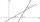Calculate the angle between these two lines: ? ?
• AngleA straight line p given by the equation ?. Calculate the size of angle in degrees between line p and y-axis.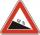Road sign informs the gradient is 10.9%. Calculate the angle which average decreases.
• The angle of lines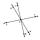Calculate the angle of two lines y=x-21 and y=-2x+14
• Hole's angles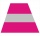I am trying to find an angle. The top of the hole is .625” and the bottom of the hole is .532”. The hole depth is .250” what is the angle of the hole (and what is the formula)?
• Cube - anglesCalculate angle between the wall diagonal and cube base. Calculate the angle between the cube body diagonal and cube base.
• Traffic signThere is a traffic sign for climbing on the road with an angle of 7%. Calculate at what angle the road rises (falls).
• BevelI have bevel in the ratio 1:6. What is the angle and how do I calculate it?
• ClimbRoad has climbing 1:27. How big is a angle corresponds to this climbing?On a straight stretch of road is marked 12 percent drop. What angle makes the direction of the road with the horizontal plane?
• PyramidPyramid has a base a = 3cm and height in v = 15 cm. a) calculate angle between plane ABV and base plane b) calculate angle between opposite side edges.Average climb of the road is given by ratio 1:15. By what angle road average climb?
• Resultant forceCalculate mathematically and graphically the resultant of a three forces with a common centre if: F1 = 50 kN α1 = 30° F2 = 40 kN α2 = 45° F3 = 40 kN α3 = 25°
• RiverFrom the observatory 11 m high and 24 m from the riverbank, river width appears in the visual angle φ = 13°. Calculate the width of the river.
• StairwayWhat angle rising stairway if step height in 20 cm and width 26 cm?
• Church tower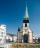Archdeacon church in Usti nad Labem has diverted tower by 186 cm. The tower is 65 m high. Calculate the angle by which the tower is tilted. Result write in degree's minutes.
• Ratio iso triangleThe ratio of the sides of an isosceles triangle is 7:6:7 Find the base angle to the nearest answer correct to 3 significant figure.
• Tangents to ellipseFind the magnitude of the angle at which the ellipse x2 + 5 y2 = 5 is visible from the point P[5, 1] .
• ConeThe rotating cone volume is 9.42 cm3, with a height 10 cm. What angle is between the side of the cone and its base?The regular quadrilateral pyramid has a base edge a = 1.56 dm and a height h = 2.05 dm. Calculate: a) the deviation angle of the sidewall plane from the base plane b) deviation angle of the side edge from the plane of the base